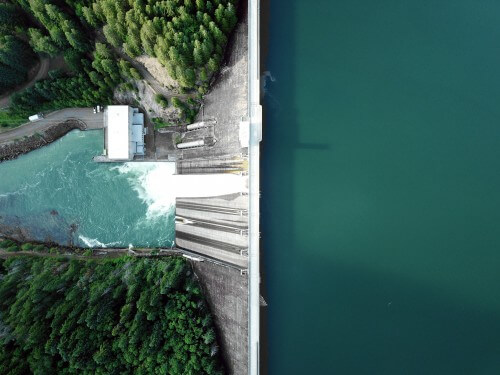# Gravity Dam – Hydraulic Structure

15th July 2019

## Gravity Dam

It is constructed with masonry or concrete it resists the force acting on it by its own weight.

### Where Gravity Dam Are Constructed?

• Good soil area
• For large spillway
• In granite basalt area
• For large height and small length dam
• For long time

### Forces Acting On Gravity Dam

Following are the 6 major forces which acting on the dam

#### 1. Weight of Dam

The total weight of the dam acts at the C.G of its section.

#### 2. Water Pressure

The horizontal water pressure P = wh²/2, this acts at height h/3 from the base of the dam.

#### 3. Pressure Due to Earth Quak

In this case two types of pressure acts

• Horizontal acceleration force FH = W.α
• Vertical acceleration force Fv = W(1- α)

(Where W = weight of dam and α = acceleration)

#### 4. Uplift Pressure

The uplift pressure is an upward pressure of water as it flows through the body of the dam or its foundation.

• Uplift pressure at the heel, A = wh
• Uplift pressure at the toe, F = wh՛
• Uplift pressure at gallery = w[h + 1/3(h-h՛)]

### Mode of Failure of Gravity Dam

1. By overturning
2. By rupture from tension
3. By sliding
4. For crushing by compressing
5. By uplift of floatation

### The Force That Gives Stability To The Dam

1. Self-weight of the dam
2. Trust of the tail water